# Electric Potential of a Dipole

## Potential due to an electric dipole

• We already know that electric dipole is an arrangement which consists of two equal and opposite charges +q and -q separated by a small distance 2a.
• Electric dipole moment is represented by a vector p of magnitude 2qa and this vector points in direction from -q to +q.
• To find electric potential due to a dipole consider charge -q is placed at point P and charge +q is placed at point Q as shown below in the figure.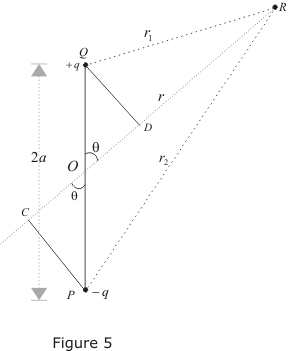• Since electric potential obeys superposition principle so potential due to electric dipole as a whole would be sum of potential due to both the charges +q and -q. Thus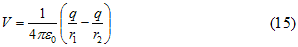where r1 and r2 respectively are distance of charge +q and -q from point R.
• Now draw line PC perpandicular to RO and line QD perpandicular to RO as shown in figure. From triangle POC
cosθ=OC/OP = OC/a
therefore OC=acosθ similarly OD=acosθ
Now ,
r1 = QR≅RD = OR-OD = r-acosθ
r2 = PR≅RC = OR+OC = r+acosθ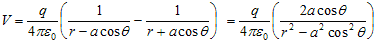since magnitude of dipole is
|p| = 2qa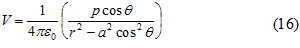• If we consider the case where r>>a then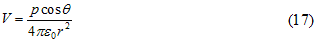again since pcosθ= p· where, is the unit vector along the vector OR then electric potential of dipole is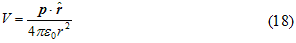for r>>a
• From above equation we can see that potential due to electric dipole is inversly proportional to r2 not 1/r which is the case for potential due to single charge.
• Potential due to electric dipole does not only depends on r but also depends on angle between position vector r and dipole moment p.

Question 1
What is the electric potential due to an electric dipole at an equatorial point?
Solution
Zero, as potential on equatorial point, due to charges of electric dipole, are equal in magnitude but opposite in nature and hence their resultant is zero.

Question 2
A short electric dipole has dipole moment of $4 \times 10^{-9}$ Cm. Find the following
(a) Electric Potential at a point distanct .3m from center of the dipole on the axial line
(b) Electric Potential at a point distanct 1 m from center of the dipole on the equatorial line
(c) Electric Potential at a point distanct .3m from center of the dipole on an line making at angle 30° with the dipole axis
Solution
Given
p=$4 \times 10^{-9}$ Cm
Potential of diple is given as
$V= \frac {1}{4 \pi \epsilon _0} \frac {p cos \theta}{r^2}$
(a) $\theta =0^0$, r=.3m
$V= \frac {1}{4 \pi \epsilon _0} \frac {p}{r^2}= 400 V$
(b) $\theta =90^0$, r=1m
$V=0$
(c) $\theta =30^0$, r=.3m
$V= \frac {1}{4 \pi \epsilon _0} \frac {p cos \theta}{r^2}$
Subsituting the values
$V=200 \sqrt 3$ V